Download Presentation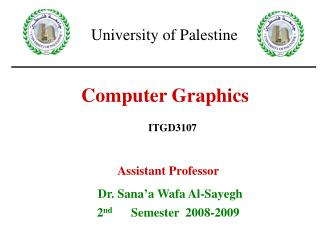Computer Graphics

# Computer Graphics - PowerPoint PPT Presentation

Assistant Professor Dr. Sana’a Wafa Al-Sayegh 2 nd Semester 2008-2009. Computer Graphics. University of Palestine. ITGD3107. ITGD3107 Computer Graphics. Chapter 3 Output Primitives line, circle , Ellipse algorithms and others. Output Primitives. What Kind of Math do We Need?I am the owner, or an agent authorized to act on behalf of the owner, of the copyrighted work described.
Download Presentation## Computer Graphics

An Image/Link below is provided (as is) to download presentation

Download Policy: Content on the Website is provided to you AS IS for your information and personal use and may not be sold / licensed / shared on other websites without getting consent from its author.While downloading, if for some reason you are not able to download a presentation, the publisher may have deleted the file from their server.

- - - - - - - - - - - - - - - - - - - - - - - - - - E N D - - - - - - - - - - - - - - - - - - - - - - - - - -
Presentation Transcript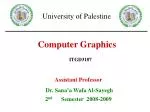Assistant Professor

Dr. Sana’a Wafa Al-Sayegh

2nd Semester 2008-2009

Computer Graphics

University of Palestine

ITGD3107

Lecture 2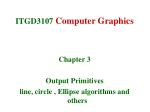ITGD3107Computer Graphics

Chapter 3

Output Primitives

line, circle , Ellipse algorithms and others

Lecture 2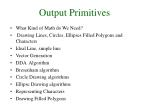Output Primitives
• What Kind of Math do We Need?
• Drawing Lines, Circles, Ellipses Filled Polygons and Characters
• Ideal Line, simple line
• Vector Generation
• DDA Algorithm
• Bresenham algorithm
• Circle Drawing algorithms
• Ellipse Drawing algorithms
• Representing Characters
• Drawing Filled Polygons

Lecture 2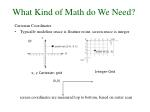What Kind of Math do We Need?

Cartesian Coordinates

Typically modeling space is floating point, screen space is integer

screen coordinates are measured top to bottom, based on raster scan

Integer Grid

x, y Cartesian grid

(0,0)

Lecture 2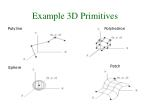Example 3D Primitives

Polyline

Polyhedron

Patch

Sphere

Lecture 2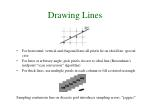Drawing Lines

For horizontal, vertical and diagonal lines all pixels lie on ideal line: special case

For lines at arbitrary angle, pick pixels closest to ideal line (Bresenham’s midpoint “scan conversion” algorithm)

For thick lines, use multiple pixels in each column or fill a rotated rectangle

Sampling continuous line on discrete grid introduces sampling errors: “jaggies”

Lecture 2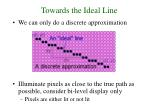Towards the Ideal Line
• We can only do a discrete approximation
• Illuminate pixels as close to the true path as possible, consider bi-level display only
• Pixels are either lit or not lit

Lecture 2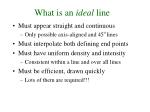What is an ideal line
• Must appear straight and continuous
• Only possible axis-aligned and 45o lines
• Must interpolate both defining end points
• Must have uniform density and intensity
• Consistent within a line and over all lines
• Must be efficient, drawn quickly
• Lots of them are required!!!

Lecture 2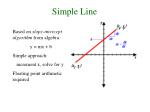Simple Line

Based on slope-intercept algorithm from algebra:

y = mx + b

Simple approach:

increment x, solve for y

Floating point arithmetic required

Lecture 2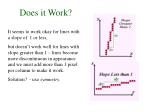Does it Work?

It seems to work okay for lines with a slope of 1 or less,

but doesn’t work well for lines with slope greater than 1 – lines become more discontinuous in appearance and we must add more than 1 pixel per column to make it work.

Solution? - use symmetry.

Lecture 2Modification

OR, increment along x-axis if dy<dx else increment along y-axis

Lecture 2Vector Generation
• There are two vector generation algorithm:
• DDA = Digital Differential Analyser
• finite differences
• Bresenham’s Algorithm

Lecture 2DDA algorithm
• DDA = Digital Differential Analyser
• finite differences
• Treat line as parametric equation in t :

Start point

End point

Lecture 2DDA Algorithm
• Start at t = 0
• At each step, increment t by dt
• Choose appropriate value for dt
• Ensure no pixels are missed:
• Implies: and
• Set dt to maximum of dx and dy

Lecture 2DDA algorithm

line(int x1, int y1, int x2, int y2)

{

float x,y;

int dx = x2-x1, dy = y2-y1;

int n = max(abs(dx),abs(dy));

float dt = n, dxdt = dx/dt, dydt = dy/dt;

x = x1;

y = y1;

while( n-- ) {

point(round(x),round(y));

x += dxdt;

y += dydt;

}

}

n - range of t.

Lecture 2DDA algorithm
• Still need a lot of floating point arithmetic.
• 2 ‘round’s and 2 adds per pixel.
• Is there a simpler way ?
• Can we use only integer arithmetic ?
• Easier to implement in hardware.

Lecture 2Bresenham (mid-point) algorithm
• Choose between 2 pixels at each step based upon the sign of a decision variable.
• Update the decision variable based upon which pixel is chosen.
• Start point is simply first endpoint (x1,y1).
• Need to calculate the initial value for d

Lecture 2Bresenham algorithmm<1

void MidpointLine1(int x1,y1,x2,y2)

{

int dx=x2-x1;

int dy=y2-y1;

int d=2*dy-dx;

int increE=2*dy;

int incrNE=2*(dy-dx);

x=x1;

y=y1;

WritePixel(x,y);

while (x < x2) {

if (d<= 0) {

d+=incrE;

x++

} else {

d+=incrNE;

x++;

y++;

}

WritePixel(x,y);

}

}

Lecture 2Bresenham algorithmm>=1

void MidpointLine2(int x1,y1,x2,y2)

{

int dx=x2-x1;

int dy=y2-y1;

int d=2*dx-dy;

int increE=2*dx;

int incrNE=2*(dx-dy);

x=x1;

y=y1;

WritePixel(x,y);

while (y < y2) {

if (d<= 0) {

d+=incrE;

y++;

} else {

d+=incrNE;

x++;

y++;

}

WritePixel(x,y);

}

}

Lecture 2Drawing Circles and Ellipses

circle outline

filled ellipse

Lecture 2Circle drawing.
• Can also use Bresenham to draw circles.
• Use 8-fold symmetry

E

M

SE

Previous

Pixel

Choices for

Next pixel

Choices for

Current pixel

Lecture 2Circle drawing.
• First method is:

to

• Where x value steps from

Lecture 2Circle drawing.
• Second method is:

Lecture 2Drawing Characters

For characters defined by small bitmap

selectively write pixels of refresh buffer corresponding to “true” bits of character bitmap

Outline fonts: defined in terms of (mathematical) drawing primitives (lines, arcs, etc).

Lecture 2Drawing Filled Polygons

Find intersection of scan line with polygon edges

Sort intersections by increasing x

Fill the polygon between pairs of intersections (spans)

Lecture 2